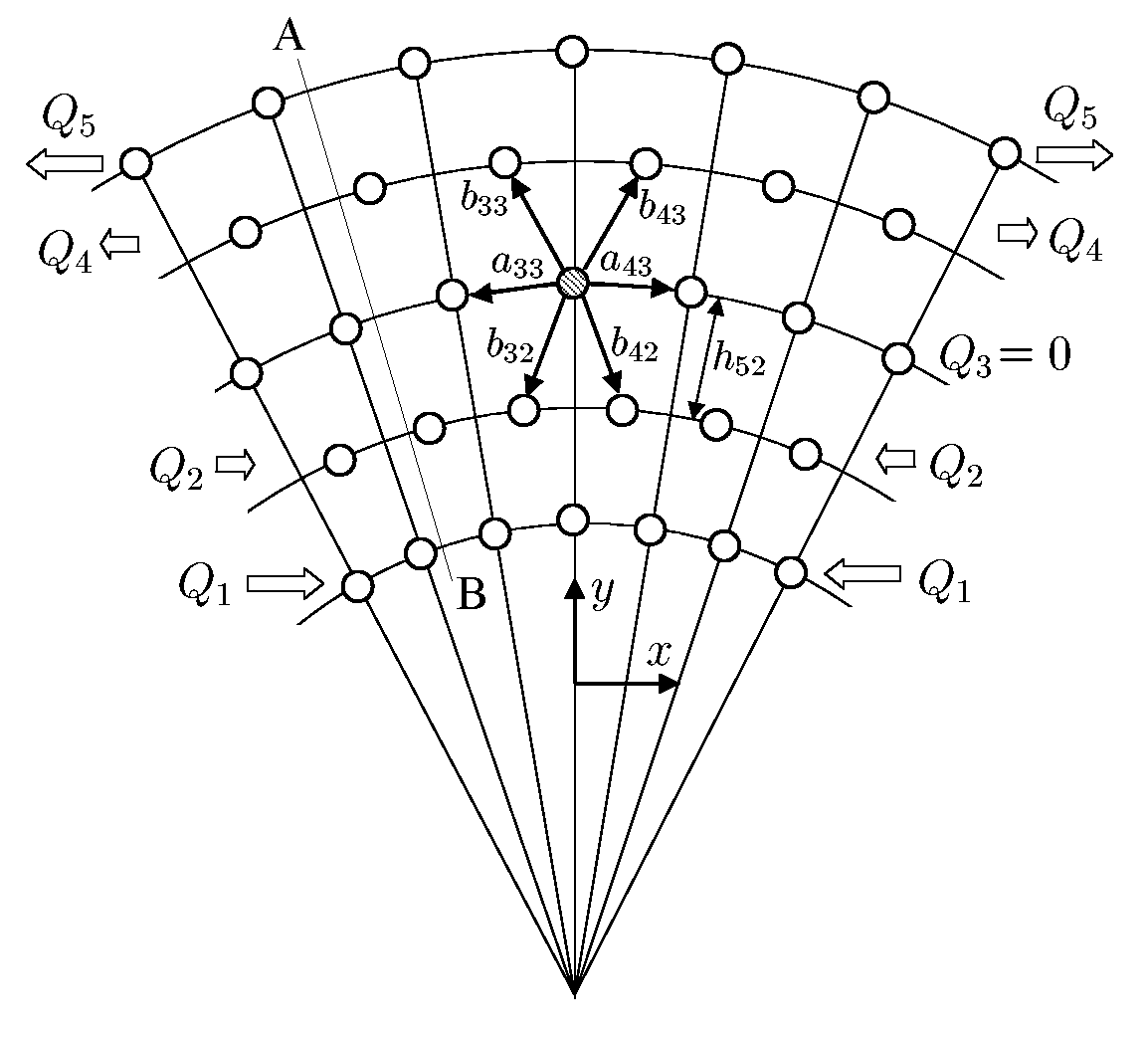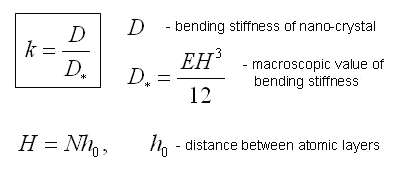Elena A. Ivanova St. Petersburg State Polytechnical UniversityMechanical properties of crystal lattices and nanocrystals

Advances in high technologies using nanometer-size structures require calculation of mechanical properties for the objects of the nano-size scale level. Majority of the theoretical mechanical models for thin-walled nano-objects, such as carbon nano-tubes, is based on the equations of shell theory. This gives the questions about applicability of the quantities obtained from the macroscopic experiments to the nanoscale objects or about necessity of corrections taking into account the scale effects. Results of theoretical investigation of interdependence of microscopic and macroscopic characteristics of nano-objects and crystal lattices are presented below. Stable models of single-layered nanostructures and crystal lattices with lower packing densities are suggested. Feature of offered models is that they are based on pairwise moment potentials of interaction.

Influence of the scale factor on bending stiffness of nano-objectsThe inconsistency of values of elastic moduli, which were obtained in microscale and macroscale experiments, was noted by many researchers. This gives the questions about necessity of corrections of values of elastic moduli, which were obtained in macroscale experiments, taking into account the scale effects. A result of theoretical investigation of the influence of the scale effects on the bending stiffness of a 2D nanocrystal (see figure) is presented on a diagram. The diagram shows dependence of dimensionless parameter k from number of atomic layers N in a direction of thickness of a nanocrystal, where k is the ratio of bending stiffness of a nanocrystal to macroscopical value of bending stiffness.Determination of bending stiffness of monolayer nano-objectsThe existence of monolayer nanotubes, which are not loaded with internal pressure, makes it necessary to consider not only the force interaction between atoms but also the moment interaction. Otherwise, the layer of atoms, forming a nanotube, would not have bending stiffness, and hence, the monolayer nanotube would be unstable. Situation here is the same, as in continual macro-mechanics. Such popular in engineering calculations continual models as rods and shells, are based on the idea of taking into account rotation degrees of freedom and the moment interactions. Ignoring of the moment interactions leads to the model of a rod degenerates in model of a filament and the model of a shell degenerates in model of a membrane. Filaments and membranes do not have bending stiffness. They are capable to keep their forms only due to external loadings, boundary conditions and stretch stiffness. A method of determining of bending stiffness of nanostructures with allowance for the moment interaction on the nanolevel has been developed by the example of discrete model of a monocrystal (see figure). We consider a crystal consisting of particles whose interaction depends not only on their relative position in space but also on their relative orientation. Interaction of the particles is characterized by the force vector and moment vector. Taking into account the moment interaction, we have obtained the corrected value of bending stiffness for nanocrysral, which is not equal to zero for monolayer nanostructure. Let us note, that influence of the moment interactions is not very essential for twolayer nanostructure and practically imperceptible for threelayer ones.

Creation of stable models of lattices with low density of packing

Pairwise interaction potentials, for example, Lennard-Jones, Mie, or Morse potentials, are widely used for modeling molecular systems. These potentials have a clear physical meaning and, although rather simple, enable one to describe the properties of a great number of substances on the qualitative level. It is well known, however, that these potentials have essential drawbacks that restrict their application area. One of the major drawbacks is that the pairwise potentials can provide stability only for crystal lattices with dense packing. This is a triangular lattice in the 2D case and face-centered cubic lattice (or one similar to that) in 3D case. 3D and 2D lattices with lower packing densities (e.g., a simple cubic lattice) are unstable for most of the pairwise potentials. This is for that reason that the distance between the second neighbors (along the diagonals of the cube) lies on the unstable segment of the force-distance diagram, i.e., an increase in the distance leads to a destabilizing, rather than restoring, force. This leads, for example, to the fact that the deformation of a square lattice into a rhombic one turns out to be energetically profitable. The structures characteristic of covalent crystals - hexagonal lattice on plane (graphite structure) and diamond structure in space - are also unstable. The traditional solution of this problem implies the utilization of many-particle interaction potentials. These potentials depend on the angles between the bonds, which enables structures with low packing density to be stabilized. On the other hand, the expressions for these potentials are rather complex and constants occurring in these expressions do not have a clear physical meaning. These constants are adjusted so as to provide the best agreement of the calculated characteristics of substances to be modeled with those known from experiments. However, when proceeding from a crystal structure to another structure (for example, from graphite to diamond), one has to completely change the interaction potential.

An alternative approach involves taking into account the torque component of the interatomic interaction. Covalent bonds are known to be directional, which enables these to transmit torque interaction. The necessity to take into account the torque interaction has been confirmed for polymers. An adequate description of the structural properties of polymer molecules is impossible without taking into account the torque interaction (corresponding to rotation about the bond direction). The torque interaction has a clear physical meaning and, for this reason, should be preferred to the many-particle interaction. By the example of a square lattice on plane (see figure), we show that by taking into account pairwise moment interaction (in addition to the pairwise force interaction) one can provide the stability of crystal structures with low packing density. Stability region for the square lattice is presented in figure.Square lattice:
a) vicinity of a selected particle,
b) variant of internal structure of a particle.If moment interaction is taken into account, then particles that are modelling atoms can not be considered as point masses. These particles, at least, should be the set of point masses rigidly connected to one another. Concrete realizations of particles models can be rather various. The only requirement to be met is that the symmetry type of the particle should be matched with that of the lattice. A criterion for selection of a model can be, on the one hand, the simplicity of this model and, on the other hand, the degree to which this model reflects the structure of the atom. In this investigation, we preferred the simplicity criterion. Particles of a general kind, forming a square lattice, are represented by set of four point masses placed at the vertices of the square and rigidly connected to one another. Each point mass interacting with point masses of another particle by means of pairwise force interaction. However, the total interaction between the particles will contain both the force and the moment components. This approach needs rather few parameters to be involved in the model. These are the parameters of the pairwise interaction and the geometrical parameters that characterize the groups of rigidly connected point masses. The model of such a kind, certainly, can not describe a structure of atom. It can be considered as model of atom only from the macroscopic point of view when the problem of construction of mechanical model of the solids containing big number of atoms is solved. To take into account the structure of the atom, one could modify the primary model as follows: One could place a point mass at the center of the square to model the nucleus and regard the point masses at the vertices of the square as electrons. In such an interpretation, it is reasonable to assume the potentials of nucleus-nucleus, nucleus-electron, and electron-electron interaction to be different. This will result in a model with a large number of parameters. This model does not possess an advantage of simplicity and, for this reason, it is difficult to assess the adequacy of this model, since by varying a large number of parameters one can always achieve good agreement with experimental data.

An alternative approach consists in modelling atoms by generic particles without specifying its structure as a set of point masses. Such a particle is called the body-point. Its position in space is set by a position vector and a turn tensor, and motion is described by two independent laws: the equation of balance of momentum and the equation of balance of angular momentum. For the first time concept of a body-point has been introduced by P.A.Zhilin. An advantage of the approach, based on modelling atoms by particles of a general kind, is that it does not imply an attempt to take into account the structure of the atom. The parameters characterizing the interaction between the generic particles are completely determined by structure of the lattices that can be formed by these particles. An implementation of this approach requires the creation of the interaction potentials that depend not only on the relative position of the particles but also on their relative orientation. In linear statement of a problem this approach is completely realized. Nonlinear statement of a problem demands the further research.

Correspondence between macroscopic and microscopic mechanical characteristics of complex crystal lattices

The mechanical model of a complex crystal lattice whose atoms are identified with the particles of a general kind possessing both translational and rotational degrees of freedom, and interacting among themselves by means of forces and the moments is suggested. In the linear theory, characteristics of atomic bonds are 2d-rank tensors of stiffness. Structure of the stiffness tensors is defined by geometry of a lattice. Moduli of elasticity should be found experimentally. Elastic properties of a lattice at a macrolevel are characterized by 4th-rank tensors of stiffness. Macroscopic stiffness tensors depend on the stiffness tensors of atomic bonds and geometry of a lattice. As a result of research it is established:

The first. Macroscopic moduli of without-moment theory of elasticity depend on both force and moment characteristics of atomic interaction. This dependence allows to determine microscopic characteristics of atomic bonds (longitudinal and cross stiffness) by moduli of elasticity of without-moment macroscopic theory. Let us emphasize, that cross stiffness of atomic bond is distinct from zero only if moment interaction of atoms is taken into account. Using characteristics of atomic bonds stiffnesses we can determine parameters of pairwise moment potentials of interaction. Pairwise moment potentials can be used as alternative of many-particle potentials in problems of computer simulating of thermomechanical processes by methods of molecular dynamics.

The second. In a layer of graphite, cross stiffness of atomic bond makes up 55 % of longitudinal one. This result shows that covalent bond is essentially noncentral one that is possible only at presence of moment interactions at a microlevel.